# Return Letters in a Front End Calculation

Hello good afternoon!

I would like some help or if it would be possible to create a calculation that would return a letter for a given situation.

Example:
if sum(d-1, 0, m1) >0 then A else 0

Only it does not return the letter A that was mentioned in the calculation.

I would like to know how I do.

1 comment
• Hi Willer

You can't return a letter as a result of a calculation - but with a small workaround, you can make it work anyhow using the number formatting.

Here's a table where I want to return an F if Profit Margin is less than 60 and maybe an A if it's above or equal to 60.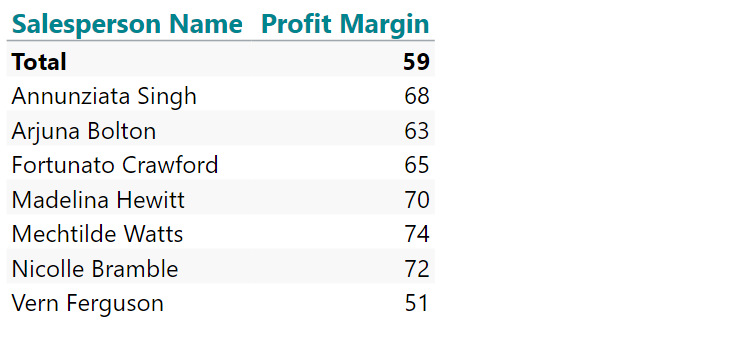My calculation syntax looks like this:
if sum(d1,0,m1)>= 60 then 1 else -1

Now my table has a calculated column (Grade):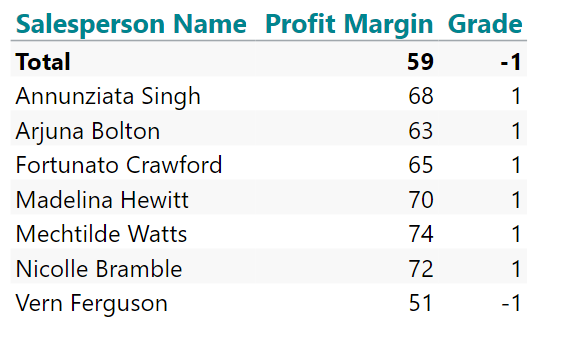Now I go to the number formatting in the properties section and choose to work with my calculated column (Grade):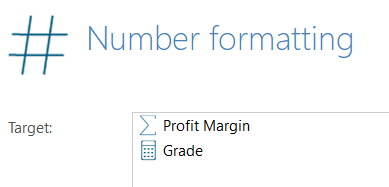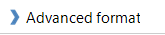In the Advanced dialogue, you can choose different formatting for positive and negative numbers: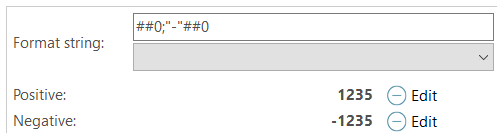The first part (until the semicolon) describes formatting for positive numbers, and after the semicolon, it's targeted at negative numbers

You can translate the mask into:
positive numbers with at least one digit;
negative number with a leading - sign and at least one digit

Now I manipulate the format string to this:

"A";"F"

That means leading A for positive numbers (and no digits) - leading F for negative numbers (and no digits).

The result: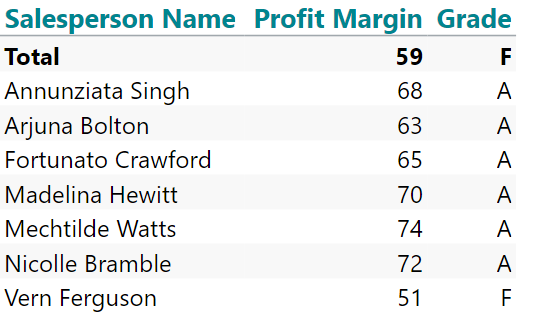Hope it makes sense

br/Niels